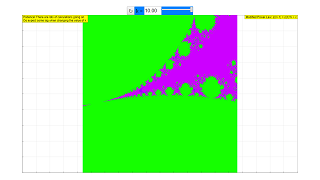## Mandelbrot Set (Variable Power) JavaScript Simulation Applet HTML5Mandelbrot Set

# Mandelbrot - set

The members of the common Mandelbrot set follow the rule

zn+1= zn2+ c ; initial value z0 = 0

c is a complex number, as is z

We look for points c in the complex plane, for which the series does not diverge. They form the Mandelbrot set. In the simulation they are colored red, lying within a range with the fractal boundary, the famous "apple man". Diverging points are colored green to blue, with the color shading indicating the speed of divergence.

Drawing a rectangle with the mouse defines a smaller range of calculation with correspondingly increasing resolution. Reset leads back to the initial condition.

Mandelbrot Set with variable power law

# Fractal for variable power law

The fractal character of the boundary of the Mandelbrot structure is a result of the nonlinearity of the series´ construction rule. It is not dependent on its specific square law rule.

To demonstrate this, a modified series is calculated with variable power law in the nonlinear term

zn+1= znk+ c; z0 = 0; k≧ 1, a rational number

c is the complex number in the plane, for which convergence or divergence of the series is determined.

The calculation is more transparent when the complex number is written in polar coordinates.

z = x + i y = r (cosφ + i sinφ) = r e

r = √(x2 + y2); φ = arctg (y / x)

z k = r k e = r k (cos (kφ) + i sin (kφ))

In the simulation a slider is used to vary the rational number k between 1 and 10. In the number field an arbitrary rational number can be input, for example an exact integer or a very high number such as 1000. k = 2 delivers the common "apple man" of the Mandelbrot set.

The default position when opening the simulation and after Reset is n = 10.

Be patient! This is a number crunching calculation, and it may take several seconds or even minutes to see the result after a change of k or after Reset, depending on the quality of your computer. When you assume that the computer has been hooked up, close the simulation and start it anew.

Experiments

E1:Choose the power k = 2 and store the contour of the "apple man" in your memory.

E2: Choose a slightly different value of k and reflect how the change originates.

E3:  Type integers 1 to 10 into the text field. Watch for symmetries, study how they relate to k, and how the structure originates.

E4: Type a very high integer for k, e.g. 1000. The fractal looks close to a circle. Why? Take a look at the boundary several times magnified.

E5: Choose integer or rational k. Use the selection rectangle of the mouse to deeply intrude into the fractal, and look for especially attractive structures. Where are they? Why? (not an easy question).

Authors

mandelbrot xlm was originally developed by

Francisco Esquembre and Wolfgang Christian The variations were developed by Dieter Röß in October 2008

This simulation is part of

– Plus 2000 Examples from Physics”

ISBN 978-3-11-025005-3, Walter de Gruyter GmbH & Co. KG

### Translations

Code Language Translator Run### Software Requirements

SoftwareRequirements

 Android iOS Windows MacOS with best with Chrome Chrome Chrome Chrome support full-screen? Yes. Chrome/Opera No. Firefox/ Samsung Internet Not yet Yes Yes cannot work on some mobile browser that don't understand JavaScript such as..... cannot work on Internet Explorer 9 and below

### CreditsDieter Roess - WEH- Foundation; Tan Wei Chiong; Loo Kang Wee; Francisco Esquembre ; Wolfgang Christian

[text]

### For Teachers

This is yet another variation of the Mandelbrot Set, the latest of the Mandelbrot series, you could say.

This time, what varies is the power to which the complex number z is raised to in each iteration.

The standard Mandelbrot Set is iterated as follows: z(n+1) = z(n)^2 + c

However, in this simulation, we define the algorithm as follows:

z(n+1) = z(n)^k + c, where k is a real number.

The value of k can be adjusted with either the slider, which allows the values to be set between 1 and 10, or with the field provided, which allows for any real value of k to be entered. This set is also called the Multibrot Set, as a portmanteau of "Multiple" and "Mandelbrot".

Disclaimer: Due to the colouring algorithm used, selecting negative powers will end up merely returning a monotone page of colour. We are currently working on implementing a better colouring algorithm for all our Mandelbrot Set simulations.
The default value of k is set to k = 10

Research

[text]

[text]

### Version:

1. http://weelookang.blogspot.sg/2016/02/vector-addition-b-c-model-with.html improved version with joseph chua's inputs
2. http://weelookang.blogspot.sg/2014/10/vector-addition-model.html original simulation by lookang

[text]

### end faq

Rating 5.00 (1 Vote)